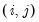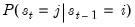switchvar
Estimate a switching VAR specification.
Syntax:
var_name.switchvar(options) lag_pairs endog_list [@ exog_list] [ @prv list_of_probability_regressors ]
You must specify the order of the VAR (using one or more pairs of lag intervals), and then provide a list of series or groups to be used as endogenous variables. You may include exogenous variables such as trends and seasonal dummies in the VAR by including an “@-sign” followed by a list of series or groups. A constant is automatically added to the list of exogenous variables; to estimate a specification without a constant, you should use the option “noconst”.
Options
General options

 msm Switching mean specification (default is switching intercept). exognv Exogenous variables are non-regime specific (default is for exogenous variables to vary). endogvary Lagged endogenous variables are regime specific (default is for the endogenous variables to be non-varying). noconst Do not include a constant in exogenous regressors list. fprobmat=arg Name of fixed transition probability matrix allows for fixing specific elements of the time-invariant transition matrix. Leave NAs in elements of the matrix to estimate. Theelement of the matrix corresponds to. initprob=arg (default=“ergodic”) Method for determining initial Markov regime probabilities: ergodic solution (“ergodic”), estimated parameter (“est”), equal probabilities (“uniform”), user-specified probabilities (“user”).If “initprob=user” is specified, you will need to specify the “userinit=” option. userinit=arg Name of vector containing user-specified initial Markov probabilities. The vector should have rows equal to the number of states; we expand this to the size of the initial lag state vector where necessary for AR specifications.For use in specifications containing both the “type=markov” and “initprob=user” options. startnum=arg (default=0 or 25) Number of random starting values tried. The default is 0 for user-supplied coefficients (option “s”) and 25 in all other cases. startiter=arg (default=10) Number of iterations taken after each random start before comparing objective to determine final starting value. searchnum=arg (default=0) Number of post-estimation perturbed starting values tried. searchsds=arg (default=1) Number of standard deviations to use in perturbed starts (if “searchnum=”) is specified. seed=positive_integer from 0 to 2,147,483,647 Seed the random number generator.If not specified, EViews will seed random number generator with a single integer draw from the default global random number generator. rnd=arg (default=“kn” or method previously set using rndseed). Type of random number generator: improved Knuth generator (“kn”), improved Mersenne Twister (“mt”), Knuth’s (1997) lagged Fibonacci generator used in EViews 4 (“kn4”) L’Ecuyer’s (1999) combined multiple recursive generator (“le”), Matsumoto and Nishimura’s (1998) Mersenne Twister used in EViews 4 (“mt4”).
In addition to the specification options, there are options for estimation and covariance calculation.